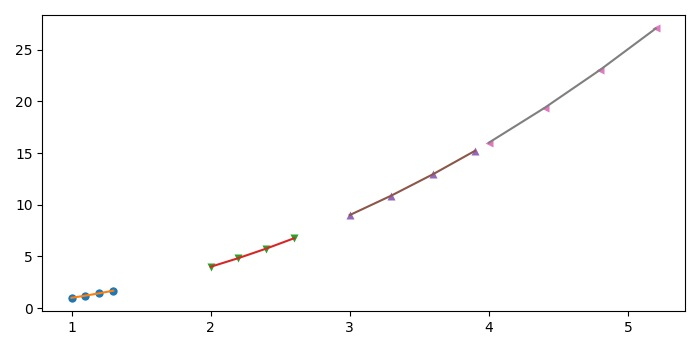# How to set same color for markers and lines in a Matplotlib plot loop?

To set the same color for markers and lines in a matplotlib, we can take the following Steps −

• Initialize m, n and x data points using numpy.

• Create a new figure or activate an existing figure using figure() method.

• Clear the figure using clf() method.

• Add a subplot to the current figure using subplot() method.

• Get a marker from a iterable marker type.

• Iterate a range from 1 to n.

• Plot the lines and markers in the loop using plot() method with the same marker and colors for a line.

• To display the figure, use show() method.

## Example

import numpy as np
import itertools
from matplotlib import pyplot as plt
plt.rcParams["figure.figsize"] = [7.00, 3.50]
plt.rcParams["figure.autolayout"] = True
m = 5
n = 5
x = np.zeros(shape=(m, n))
plt.figure()
plt.clf()
plt.subplot(111)
marker = itertools.cycle(('o', 'v', '^', '<', '>', 's', '8', 'p'))
for i in range(1, n):
x = np.dot(i, [1, 1.1, 1.2, 1.3])
y = x ** 2
plt.plot(x, y, linestyle='', markeredgecolor='none', marker=next(marker), alpha=1)
plt.plot(x, y, linestyle='-')
plt.show()

## Output